# NCERT Exemplar Class 12 Chemistry Solutions for Chapter 2 - Solutions

NCERT Class 12 Chemistry exemplar for Chapter 2 Solutions helps you focus on fundamentals to help students with basic concepts. This will make you understand the chapter 2 solutions thoroughly thereby assist you in scoring high marks in Class 12 board examination and graduate entrance examinations.

NCERT Class 12 Chemistry exemplar for chapter 2 pdf is an important study material that provides you with exemplar questions and problems given in NCERT Exemplar book. Along with NCERT questions, this pdf has several extra questions prepared by experts at BYJU’S. This solution has MCQs, fill in the blanks, match the following, exercises, assignments and numerical problems that help your exam preparation.

## Download PDF of NCERT Exemplar Solutions For Class 12 Chemistry Chapter 2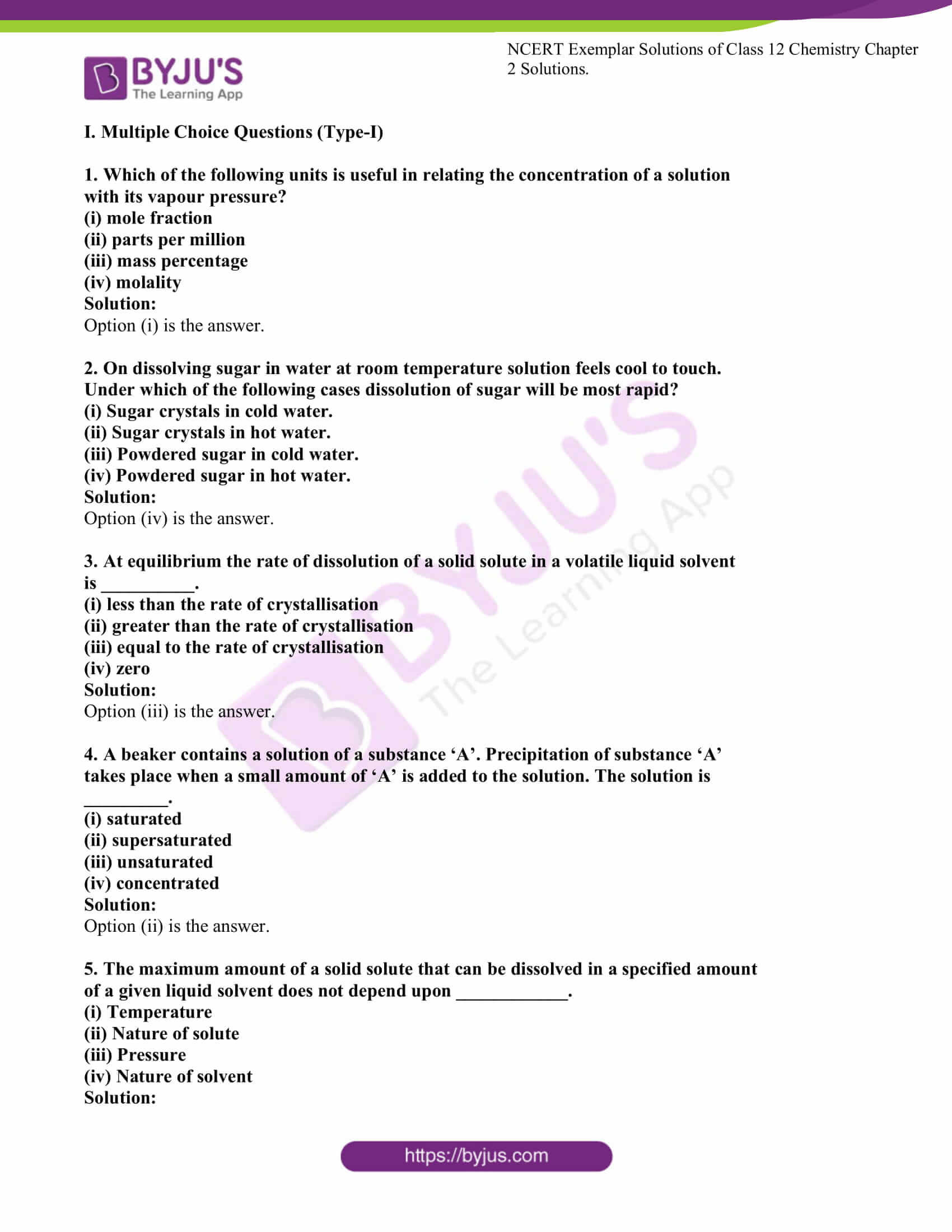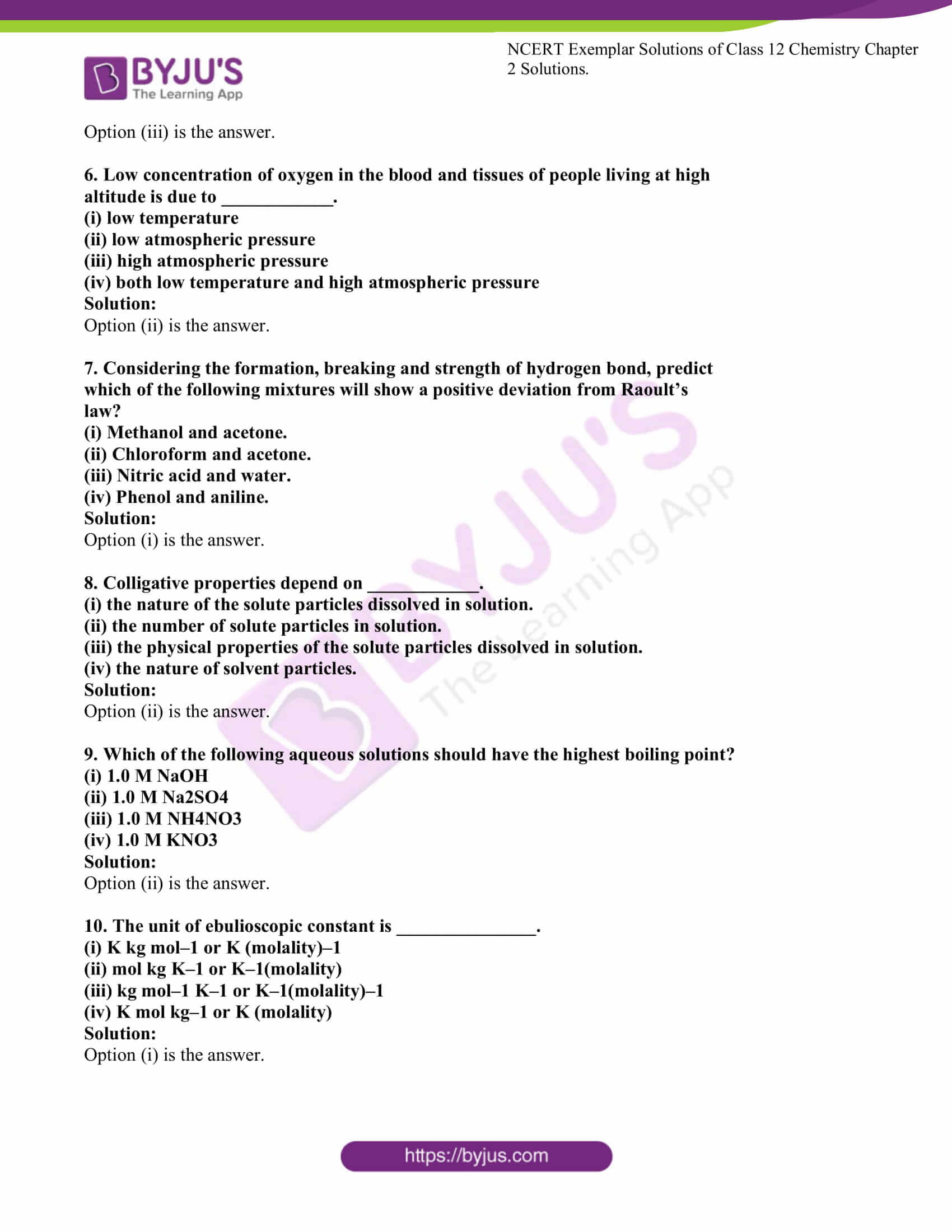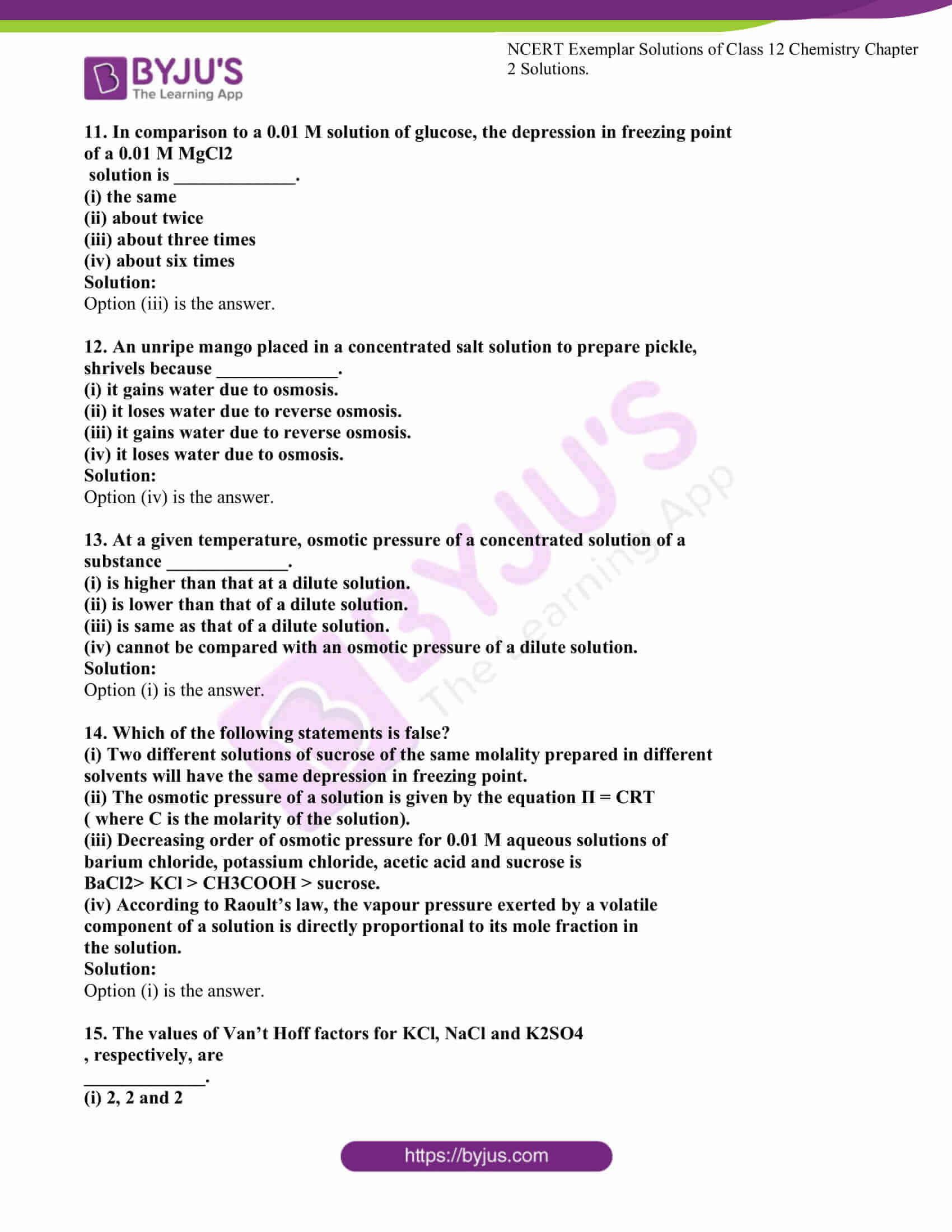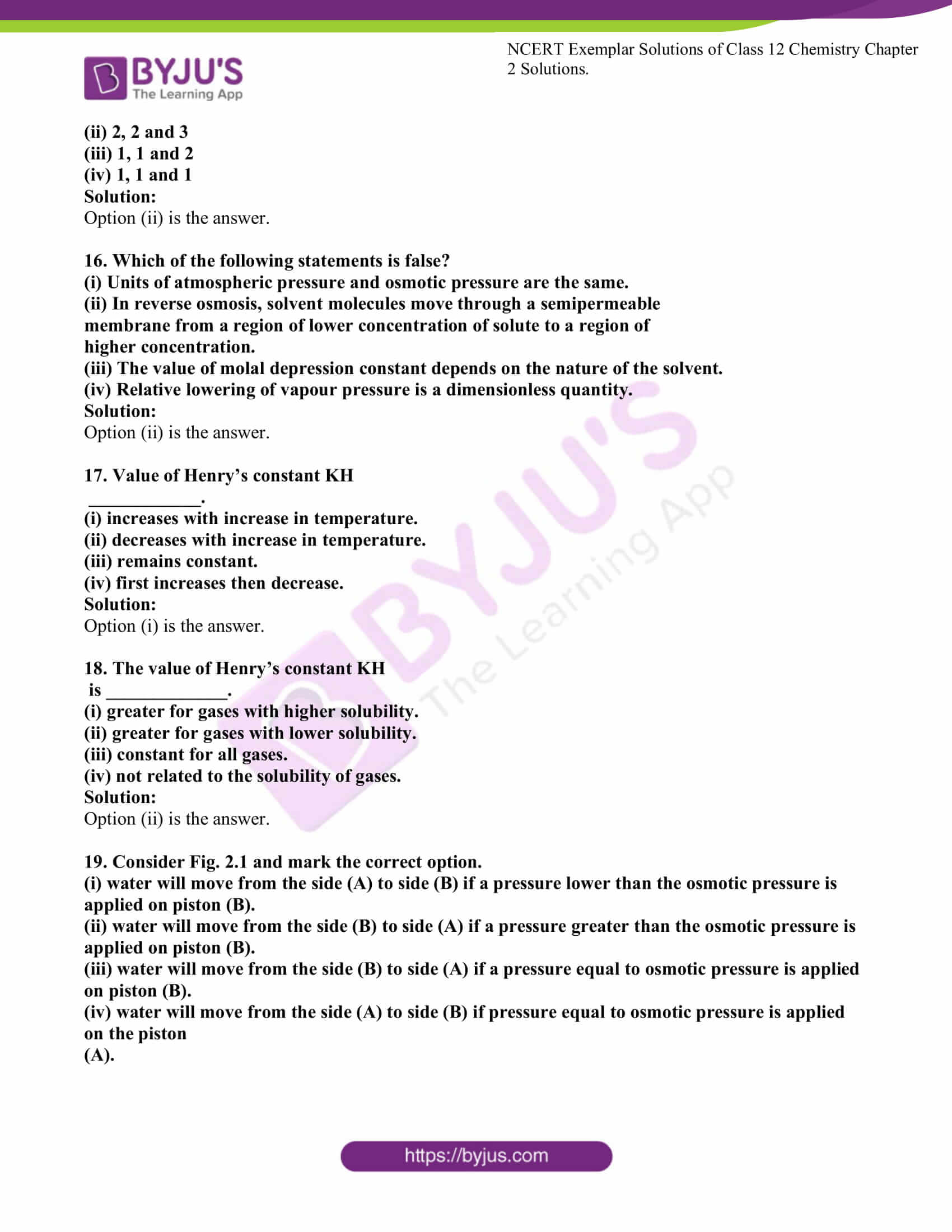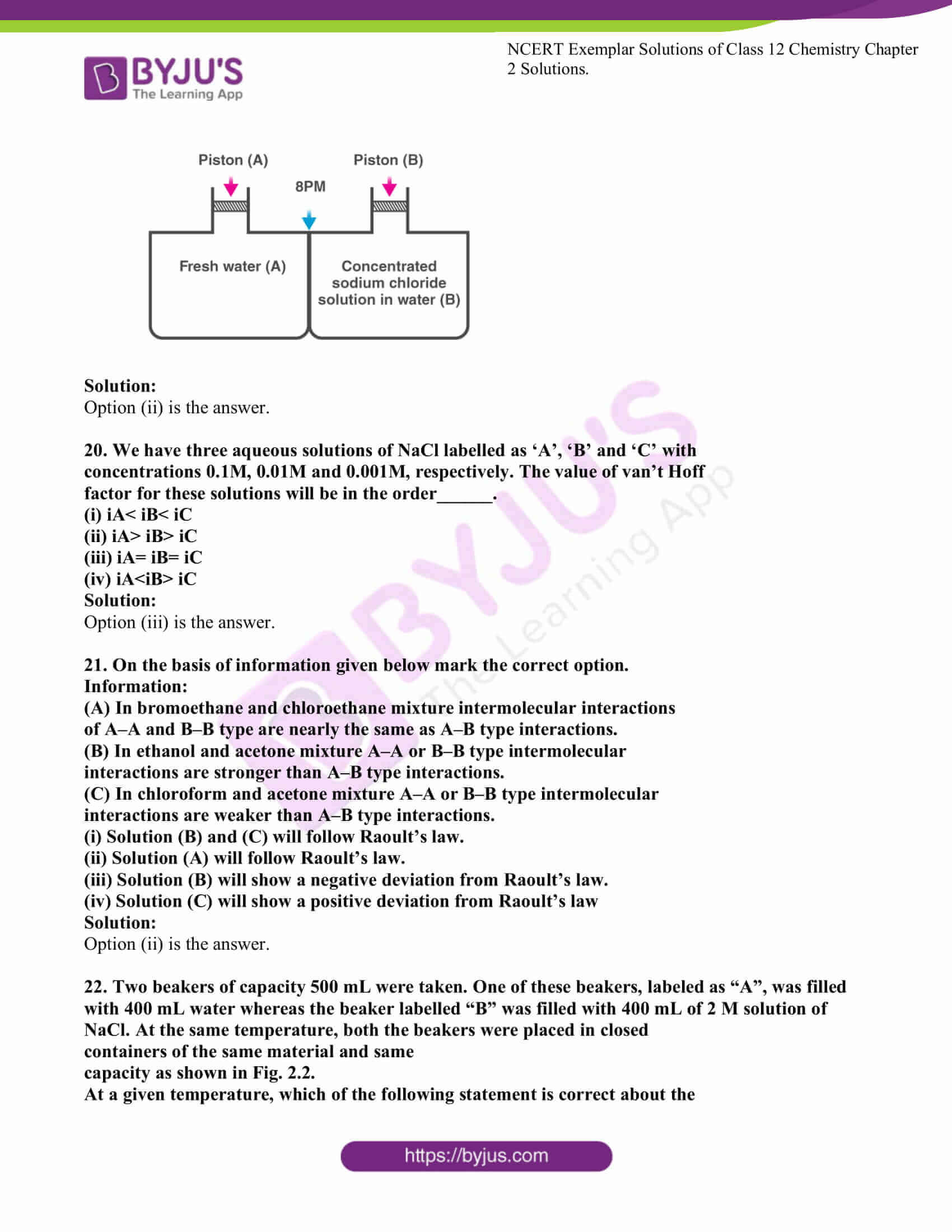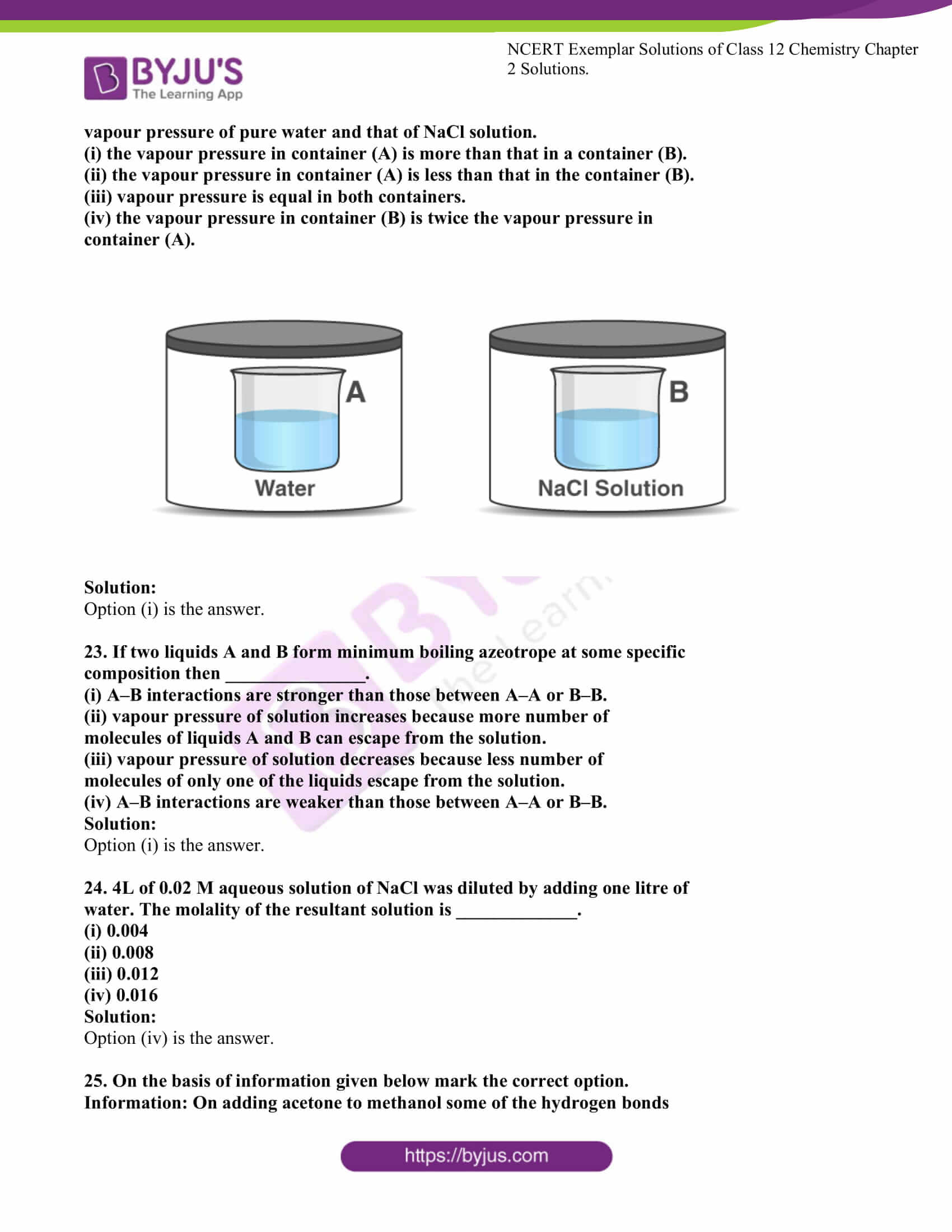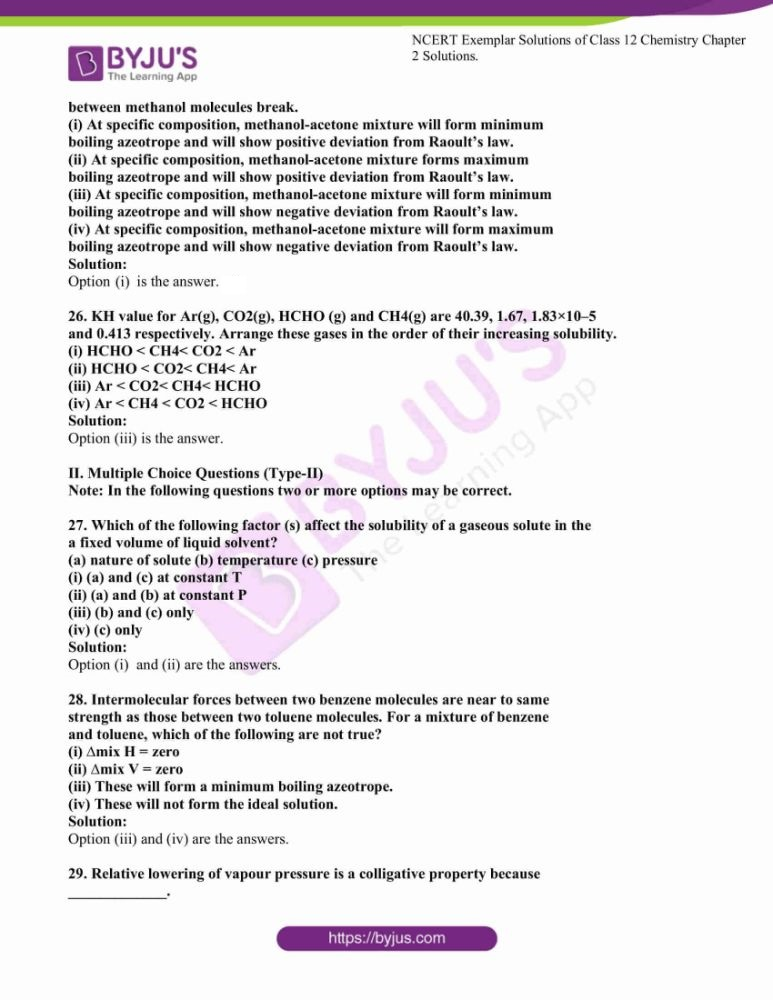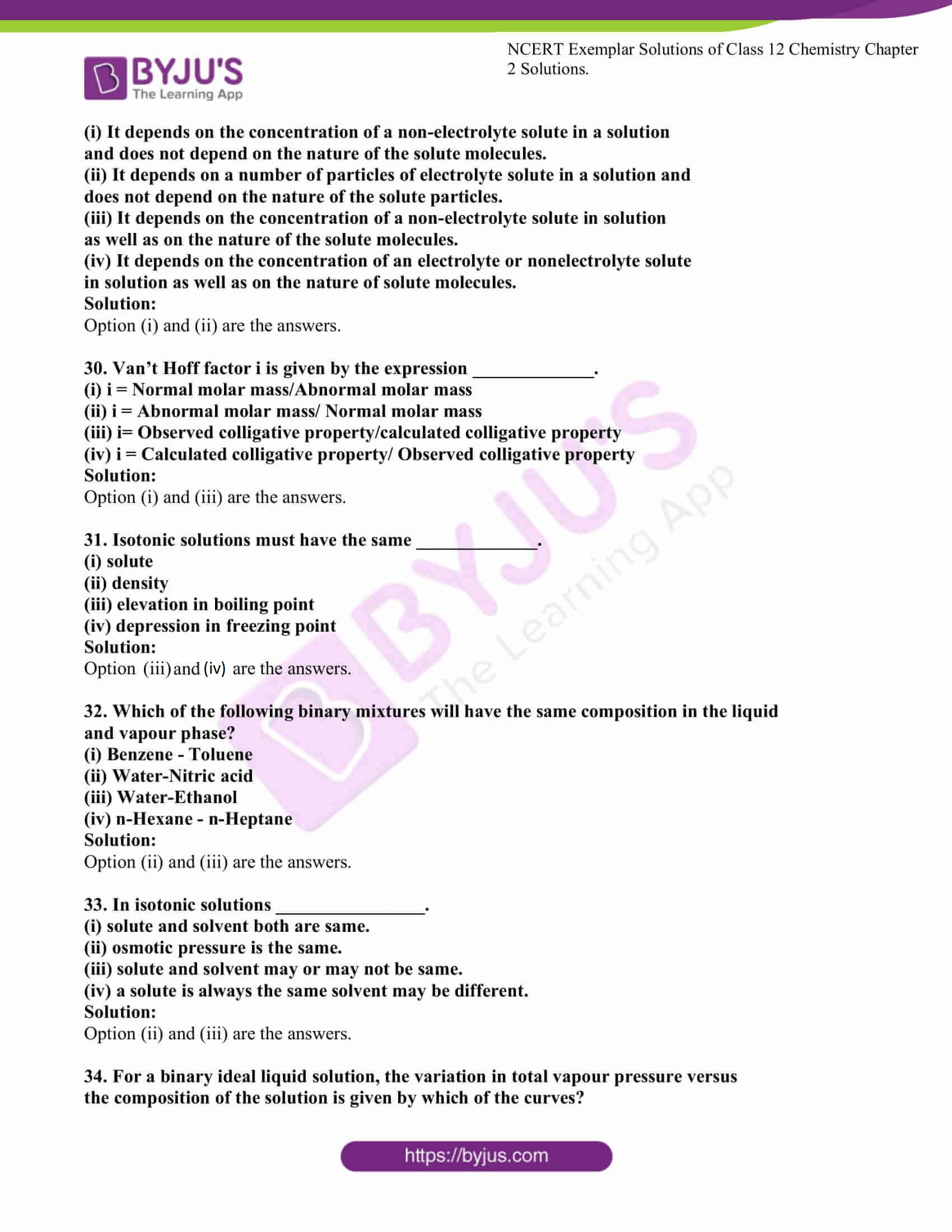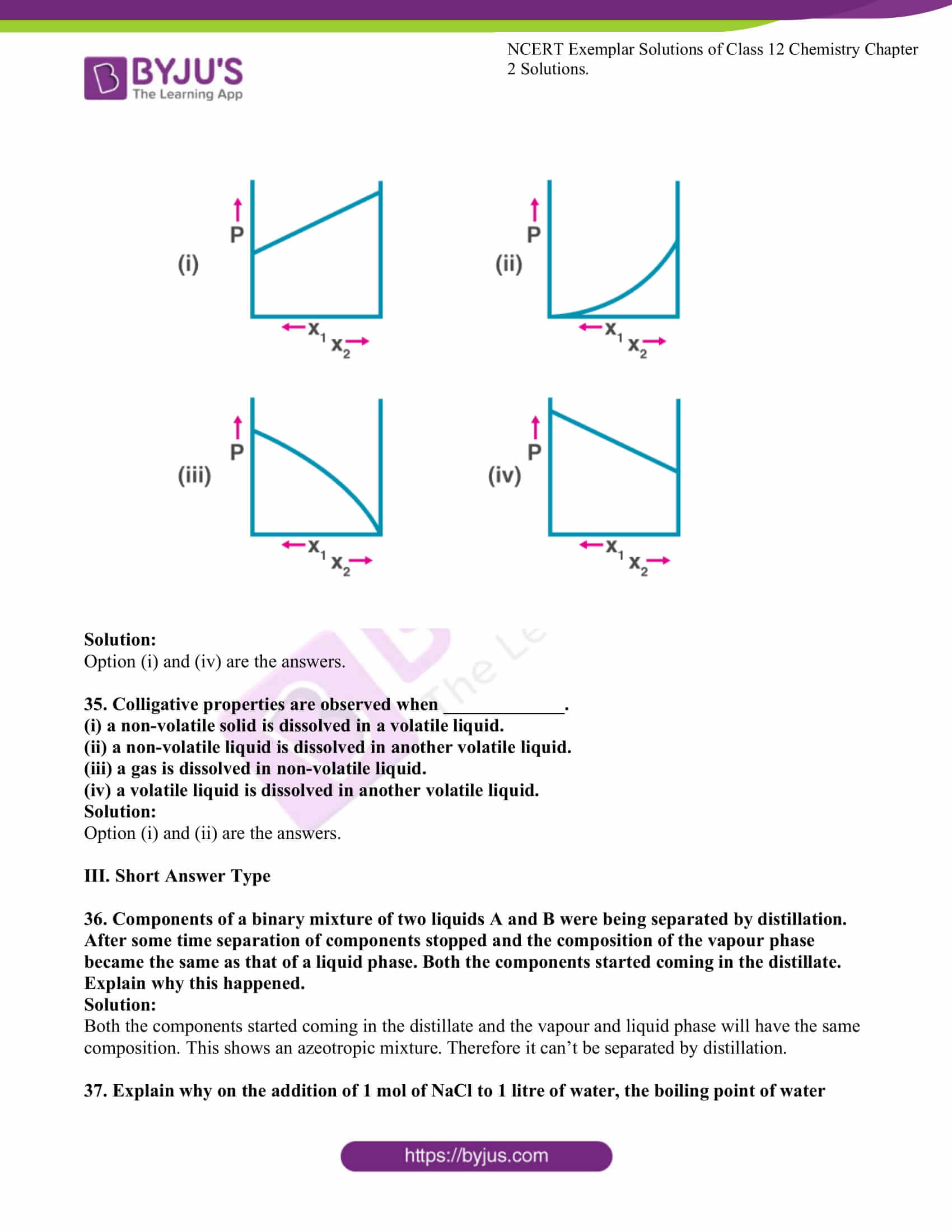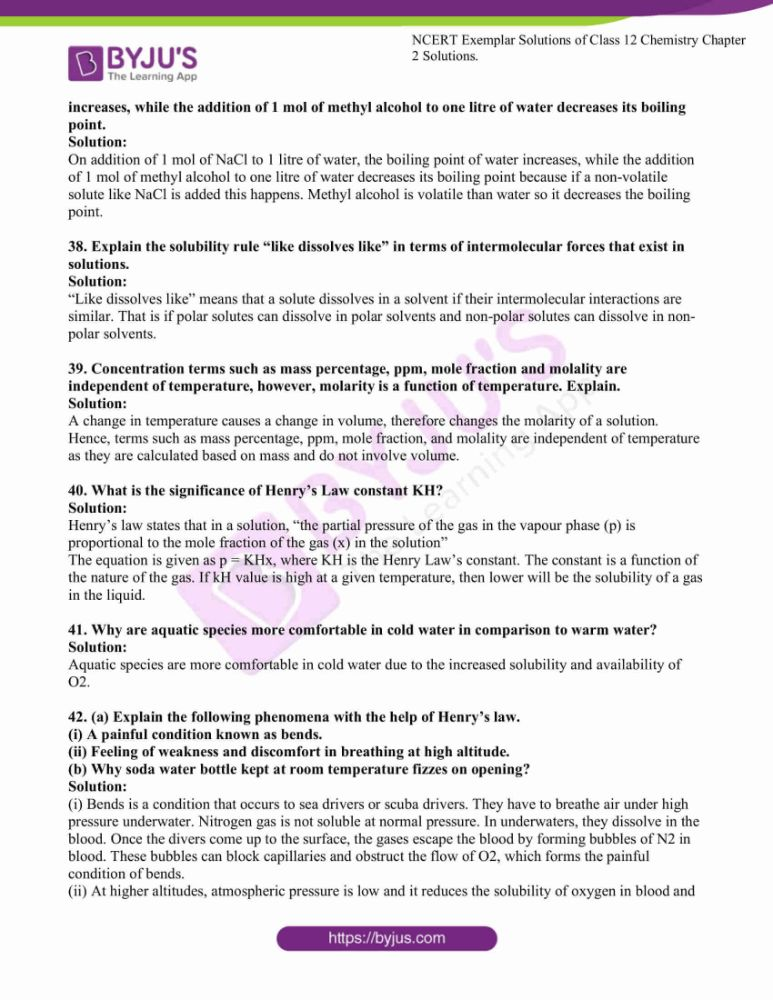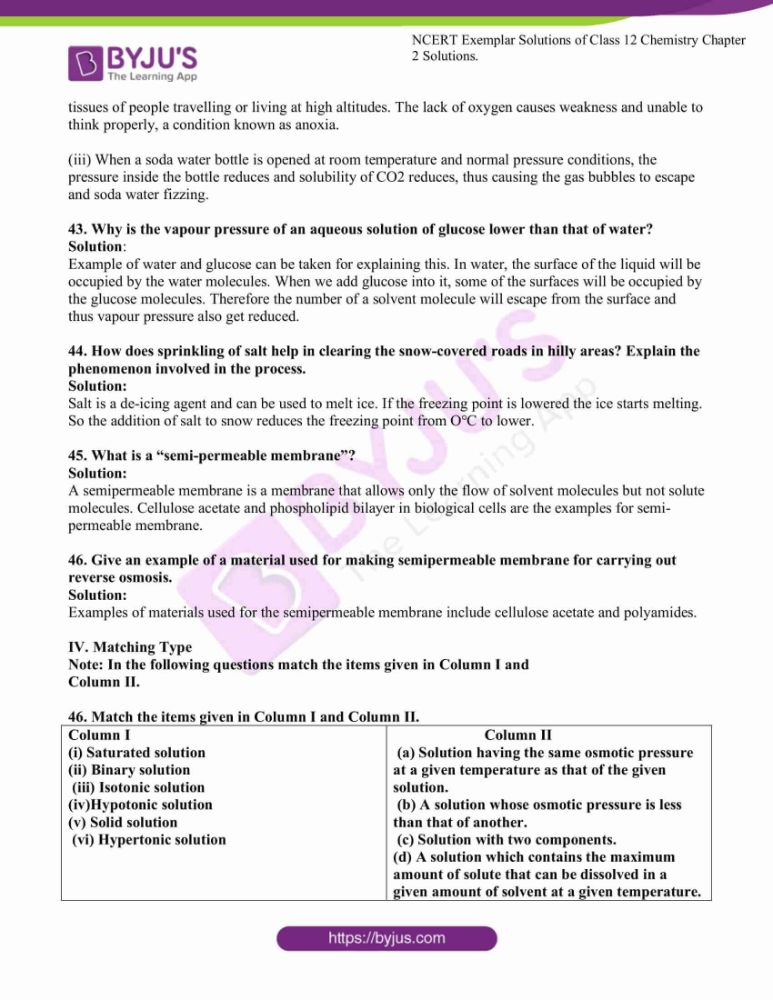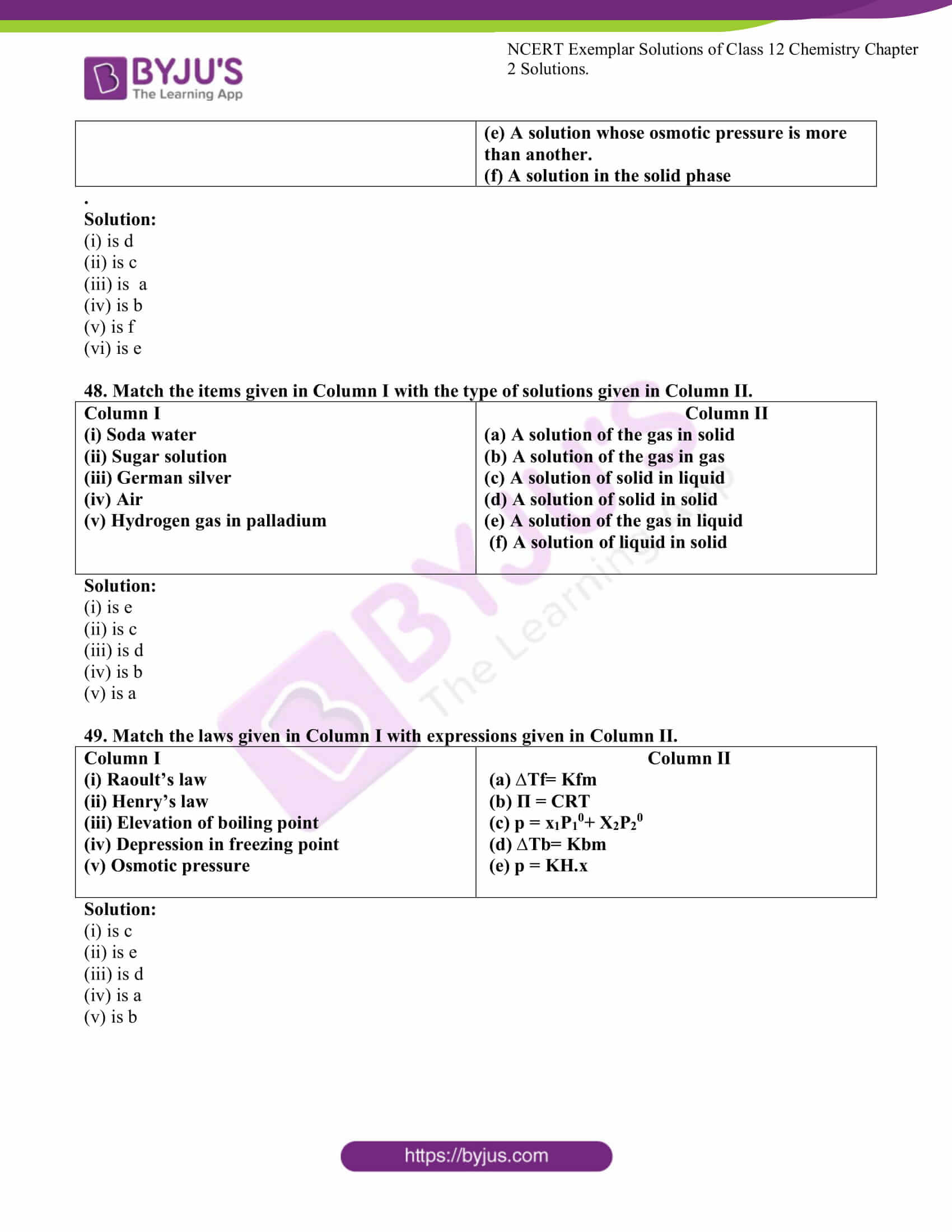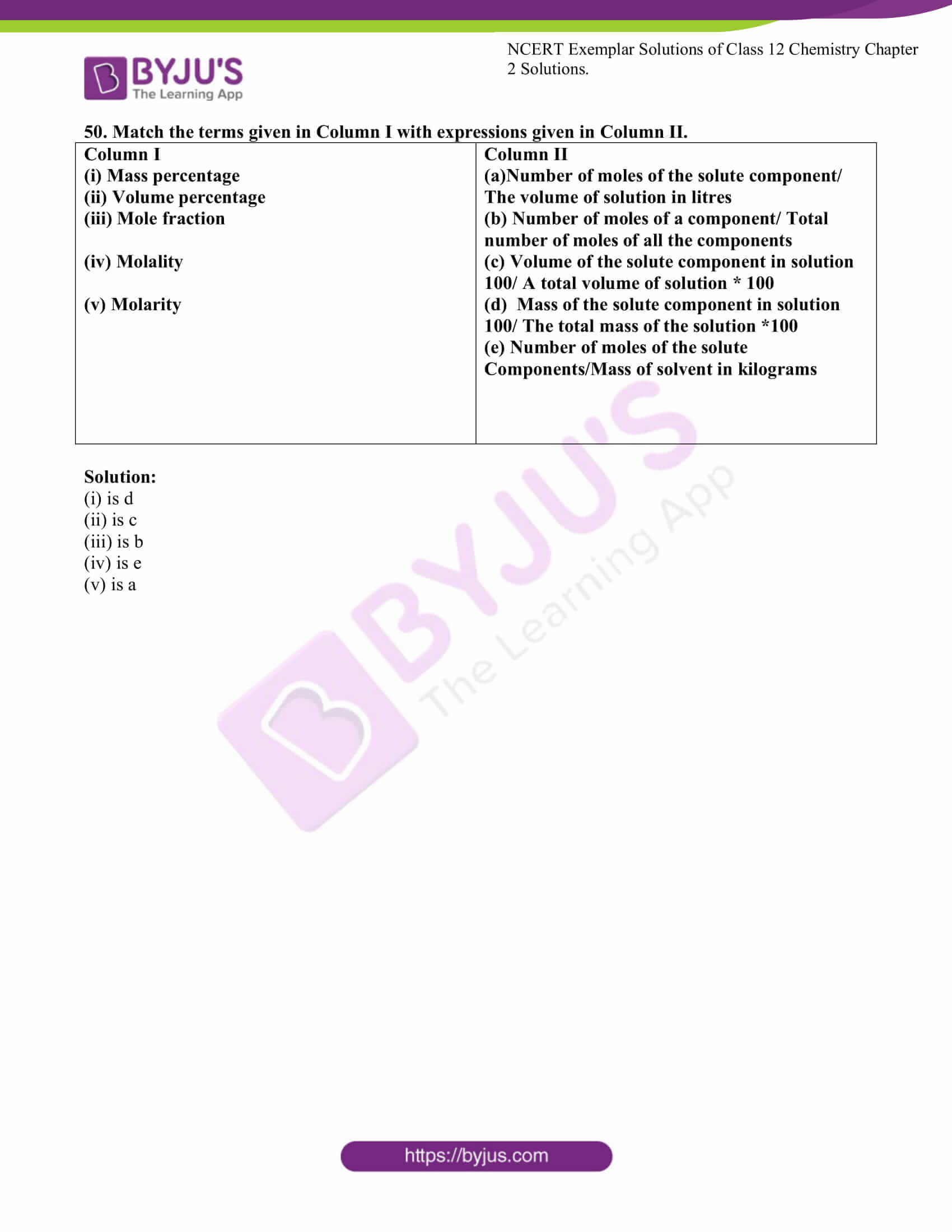### Access Solutions For NCERT Exemplar Class 12 Chemistry Chapter 2

I. Multiple Choice Questions (Type-I)

1. Which of the following units is useful in relating the concentration of a solution

with its vapour pressure?

(i) mole fraction

(ii) parts per million

(iii) mass percentage

(iv) molality

Solution:

2. On dissolving sugar in water at room temperature solution feels cool to touch.

Under which of the following cases dissolution of sugar will be most rapid?

(i) Sugar crystals in cold water.

(ii) Sugar crystals in hot water.

(iii) Powdered sugar in cold water.

(iv) Powdered sugar in hot water.

Solution:

3. At equilibrium the rate of dissolution of a solid solute in a volatile liquid solvent

is __________.

(i) less than the rate of crystallisation

(ii) greater than the rate of crystallisation

(iii) equal to the rate of crystallisation

(iv) zero

Solution:

4. A beaker contains a solution of a substance ‘A’. Precipitation of substance ‘A’

takes place when a small amount of ‘A’ is added to the solution. The solution is

_________.

(i) saturated

(ii) supersaturated

(iii) unsaturated

(iv) concentrated

Solution:

5. The maximum amount of a solid solute that can be dissolved in a specified amount

of a given liquid solvent does not depend upon ____________.

(i) Temperature

(ii) Nature of solute

(iii) Pressure

(iv) Nature of solvent

Solution:

6. Low concentration of oxygen in the blood and tissues of people living at high

altitude is due to ____________.

(i) low temperature

(ii) low atmospheric pressure

(iii) high atmospheric pressure

(iv) both low temperature and high atmospheric pressure

Solution:

7. Considering the formation, breaking and strength of hydrogen bond, predict

which of the following mixtures will show a positive deviation from Raoult’s

law?

(i) Methanol and acetone.

(ii) Chloroform and acetone.

(iii) Nitric acid and water.

(iv) Phenol and aniline.

Solution:

8. Colligative properties depend on ____________.

(i) the nature of the solute particles dissolved in solution.

(ii) the number of solute particles in solution.

(iii) the physical properties of the solute particles dissolved in solution.

(iv) the nature of solvent particles.Solution:

9. Which of the following aqueous solutions should have the highest boiling point?

(i) 1.0 M NaOH

(ii) 1.0 M Na2SO4

(iii) 1.0 M NH4NO3

(iv) 1.0 M KNO3

Solution:

10. The unit of ebulioscopic constant is _______________.

(i) K kg mol–1 or K (molality)–1

(ii) mol kg K–1 or K–1(molality)(iii) kg mol–1 K–1 or K–1(molality)–1

(iv) K mol kg–1 or K (molality)

Solution:

11. In comparison to a 0.01 M solution of glucose, the depression in freezing point

of a 0.01 M MgCl2

solution is _____________.

(i) the same

Solution:

12. An unripe mango placed in a concentrated salt solution to prepare pickle,

shrivels because _____________.

(i) it gains water due to osmosis.

(ii) it loses water due to reverse osmosis.

(iii) it gains water due to reverse osmosis.

(iv) it loses water due to osmosis.

Solution:

13. At a given temperature, osmotic pressure of a concentrated solution of a

substance _____________.

(i) is higher than that at a dilute solution.

(ii) is lower than that of a dilute solution.

(iii) is same as that of a dilute solution.

(iv) cannot be compared with an osmotic pressure of a dilute solution.

Solution:

14. Which of the following statements is false?

(i) Two different solutions of sucrose of the same molality prepared in different

solvents will have the same depression in freezing point.

(ii) The osmotic pressure of a solution is given by the equation Π = CRT

( where C is the molarity of the solution).

(iii) Decreasing order of osmotic pressure for 0.01 M aqueous solutions of

barium chloride, potassium chloride, acetic acid and sucrose is

BaCl2> KCl > CH3COOH > sucrose.

(iv) According to Raoult’s law, the vapour pressure exerted by a volatile

component of a solution is directly proportional to its mole fraction in

the solution.

Solution:

15. The values of Van’t Hoff factors for KCl, NaCl and K2SO4

, respectively, are

_____________.

(i) 2, 2 and 2

(ii) 2, 2 and 3

(iii) 1, 1 and 2

(iv) 1, 1 and 1

Solution:

16. Which of the following statements is false?

(i) Units of atmospheric pressure and osmotic pressure are the same.

(ii) In reverse osmosis, solvent molecules move through a semipermeable

membrane from a region of lower concentration of solute to a region of

higher concentration.

(iii) The value of molal depression constant depends on the nature of the solvent.

(iv) Relative lowering of vapour pressure is a dimensionless quantity.

Solution:

17. Value of Henry’s constant KH

____________.

(i) increases with increase in temperature.

(ii) decreases with increase in temperature.

(iii) remains constant.

(iv) first increases then decrease.

Solution:

18. The value of Henry’s constant KH

is _____________.

(i) greater for gases with higher solubility.

(ii) greater for gases with lower solubility.

(iii) constant for all gases.

(iv) not related to the solubility of gases.

Solution:

19. Consider Fig. 2.1 and mark the correct option.

(i) water will move from the side (A) to side (B) if a pressure lower than the osmotic pressure is applied on piston (B).

(ii) water will move from the side (B) to side (A) if a pressure greater than the osmotic pressure is applied on piston (B).

(iii) water will move from the side (B) to side (A) if a pressure equal to osmotic pressure is applied on piston (B).

(iv) water will move from the side (A) to side (B) if pressure equal to osmotic pressure is applied on the piston

(A).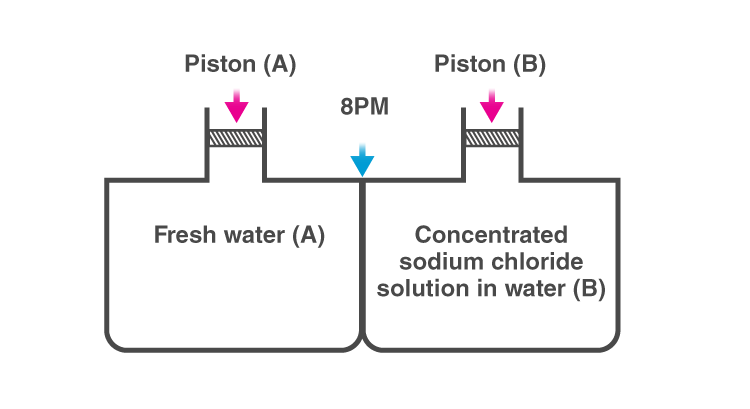Solution:

20. We have three aqueous solutions of NaCl labelled as ‘A’, ‘B’ and ‘C’ with

concentrations 0.1M, 0.01M and 0.001M, respectively. The value of van’t Hoff

factor for these solutions will be in the order______.

(i) iA< iB< iC

(ii) iA> iB> iC

(iii) iA= iB= iC

(iv) iA<iB> iC

Solution:

21. On the basis of information given below mark the correct option.

Information:

(A) In bromoethane and chloroethane mixture intermolecular interactions

of A–A and B–B type are nearly the same as A–B type interactions.

(B) In ethanol and acetone mixture A–A or B–B type intermolecular

interactions are stronger than A–B type interactions.

(C) In chloroform and acetone mixture A–A or B–B type intermolecular

interactions are weaker than A–B type interactions.

(i) Solution (B) and (C) will follow Raoult’s law.

(ii) Solution (A) will follow Raoult’s law.

(iii) Solution (B) will show a negative deviation from Raoult’s law.

(iv) Solution (C) will show a positive deviation from Raoult’s law

Solution:

22. Two beakers of capacity 500 mL were taken. One of these beakers, labeled as “A”, was filled with 400 mL water whereas the beaker labelled “B” was filled with 400 mL of 2 M solution of NaCl. At the same temperature, both the beakers were placed in closed

containers of the same material and same

capacity as shown in Fig. 2.2.

At a given temperature, which of the following statement is correct about the

vapour pressure of pure water and that of NaCl solution.

(i) the vapour pressure in container (A) is more than that in a container (B).

(ii) the vapour pressure in container (A) is less than that in the container (B).

(iii) vapour pressure is equal in both containers.

(iv) the vapour pressure in container (B) is twice the vapour pressure in

container (A).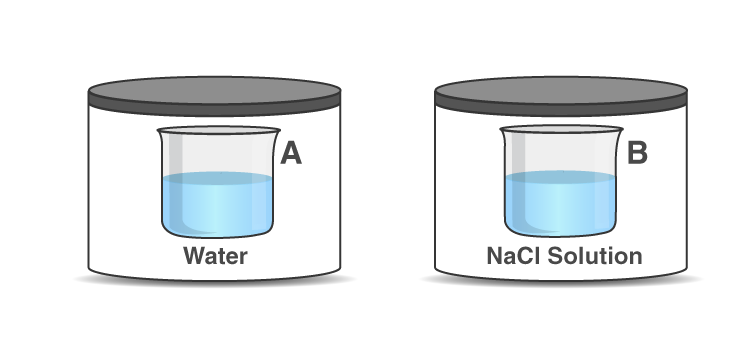Solution:

23. If two liquids A and B form minimum boiling azeotrope at some specific

composition then _______________.

(i) A–B interactions are stronger than those between A–A or B–B.

(ii) vapour pressure of solution increases because more number of

molecules of liquids A and B can escape from the solution.

(iii) vapour pressure of solution decreases because less number of

molecules of only one of the liquids escape from the solution.

(iv) A–B interactions are weaker than those between A–A or B–B.

Solution:

24. 4L of 0.02 M aqueous solution of NaCl was diluted by adding one litre of

water. The molality of the resultant solution is _____________.

(i) 0.004

(ii) 0.008

(iii) 0.012

(iv) 0.016Solution:

25. On the basis of information given below mark the correct option.

Information: On adding acetone to methanol some of the hydrogen bonds

between methanol molecules break.

(i) At specific composition, methanol-acetone mixture will form minimum

boiling azeotrope and will show positive deviation from Raoult’s law.

(ii) At specific composition, methanol-acetone mixture forms maximum

boiling azeotrope and will show positive deviation from Raoult’s law.

(iii) At specific composition, methanol-acetone mixture will form minimum

boiling azeotrope and will show negative deviation from Raoult’s law.

(iv) At specific composition, methanol-acetone mixture will form maximum

boiling azeotrope and will show negative deviation from Raoult’s law.

Solution:

26. KH value for Ar(g), CO2(g), HCHO (g) and CH4(g) are 40.39, 1.67, 1.83×10–5

and 0.413 respectively. Arrange these gases in the order of their increasing solubility.

(i) HCHO < CH4< CO2 < Ar

(ii) HCHO < CO2< CH4< Ar

(iii) Ar < CO2< CH4< HCHO

(iv) Ar < CH4 < CO2 < HCHOSolution:

II. Multiple Choice Questions (Type-II)

Note: In the following questions two or more options may be correct.

27. Which of the following factor (s) affect the solubility of a gaseous solute in the

a fixed volume of liquid solvent?

(a) nature of solute (b) temperature (c) pressure

(i) (a) and (c) at constant T

(ii) (a) and (b) at constant P

(iii) (b) and (c) only

(iv) (c) only

Solution:

Option (i) and (ii) are the answers.

28. Intermolecular forces between two benzene molecules are near to same

strength as those between two toluene molecules. For a mixture of benzene

and toluene, which of the following are not true?

(i) ∆mix H = zero

(ii) ∆mix V = zero

(iii) These will form a minimum boiling azeotrope.

(iv) These will not form the ideal solution.

Solution:

Option (iii) and (iv) are the answers.

29. Relative lowering of vapour pressure is a colligative property because

_____________.

(i) It depends on the concentration of a non-electrolyte solute in a solution

and does not depend on the nature of the solute molecules.

(ii) It depends on a number of particles of electrolyte solute in a solution and

does not depend on the nature of the solute particles.

(iii) It depends on the concentration of a non-electrolyte solute in solution

as well as on the nature of the solute molecules.

(iv) It depends on the concentration of an electrolyte or nonelectrolyte solute

in solution as well as on the nature of solute molecules.

Solution:

Option (i) and (ii) are the answers.

30. Van’t Hoff factor i is given by the expression _____________.

(i) i = Normal molar mass/Abnormal molar mass

(ii) i = Abnormal molar mass/ Normal molar mass

(iii) i= Observed colligative property/calculated colligative property

(iv) i = Calculated colligative property/ Observed colligative property

Solution:

Option (i) and (iii) are the answers.

31. Isotonic solutions must have the same _____________.

(i) solute

(ii) density

(iii) elevation in boiling point

(iv) depression in freezing point

Solution:

Option (iii) and (iv) are the answers.

32. Which of the following binary mixtures will have the same composition in the liquid

and vapour phase?

(i) Benzene – Toluene

(ii) Water-Nitric acid

(iii) Water-Ethanol

(iv) n-Hexane – n-Heptane

Solution:

Option (ii) and (iii) are the answers.

33. In isotonic solutions ________________.

(i) solute and solvent both are same.

(ii) osmotic pressure is the same.

(iii) solute and solvent may or may not be same.

(iv) a solute is always the same solvent may be different.

Solution:

Option (ii) and (iii) are the answers.

34. For a binary ideal liquid solution, the variation in total vapour pressure versus

the composition of the solution is given by which of the curves?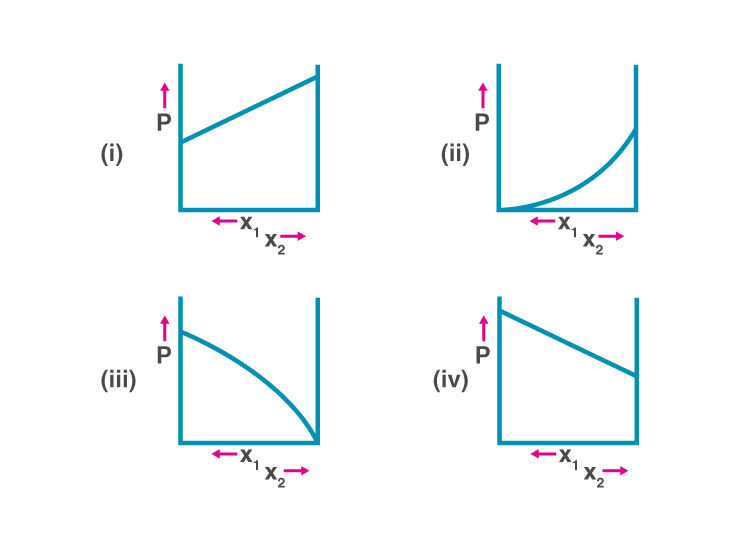Solution:

Option (i) and (iv) are the answers.

35. Colligative properties are observed when _____________.

(i) a non-volatile solid is dissolved in a volatile liquid.

(ii) a non-volatile liquid is dissolved in another volatile liquid.

(iii) a gas is dissolved in non-volatile liquid.

(iv) a volatile liquid is dissolved in another volatile liquid.

Solution:

Option (i) and (ii) are the answers.

36. Components of a binary mixture of two liquids A and B were being separated by distillation. After some time separation of components stopped and the composition of the vapour phase became the same as that of a liquid phase. Both the components started coming in the distillate. Explain why this happened.

Solution:

Both the components started coming in the distillate and the vapour and liquid phase will have the same composition. This shows an azeotropic mixture. Therefore it can’t be separated by distillation.

37. Explain why on the addition of 1 mol of NaCl to 1 litre of water, the boiling point of water increases, while the addition of 1 mol of methyl alcohol to one litre of water decreases its boiling point.

Solution:

On addition of 1 mol of NaCl to 1 litre of water, the boiling point of water increases, while the addition of 1 mol of methyl alcohol to one litre of water decreases its boiling point because if a non-volatile solute like NaCl is added this happens. Methyl alcohol is volatile than water so it decreases the boiling point.

38. Explain the solubility rule “like dissolves like” in terms of intermolecular forces that exist in solutions.

Solution:

“Like dissolves like” means that a solute dissolves in a solvent if their intermolecular interactions are similar. That is if polar solutes can dissolve in polar solvents and non-polar solutes can dissolve in non-polar solvents.

39. Concentration terms such as mass percentage, ppm, mole fraction and molality are independent of temperature, however, molarity is a function of temperature. Explain.

Solution:

A change in temperature causes a change in volume, therefore changes the molarity of a solution. Hence, terms such as mass percentage, ppm, mole fraction, and molality are independent of temperature as they are calculated based on mass and do not involve volume.

40. What is the significance of Henry’s Law constant KH?

Solution:

Henry’s law states that in a solution, “the partial pressure of the gas in the vapour phase (p) is proportional to the mole fraction of the gas (x) in the solution”

The equation is given as p = KHx, where KH is the Henry Law’s constant. The constant is a function of the nature of the gas. If kH value is high at a given temperature, then lower will be the solubility of a gas in the liquid.

41. Why are aquatic species more comfortable in cold water in comparison to warm water?

Solution:

Aquatic species are more comfortable in cold water due to the increased solubility and availability of O2.

42. (a) Explain the following phenomena with the help of Henry’s law.

(i) A painful condition known as bends.

(ii) Feeling of weakness and discomfort in breathing at high altitude.

(b) Why soda water bottle kept at room temperature fizzes on opening?

Solution:

(i) Bends is a condition that occurs to sea drivers or scuba drivers. They have to breathe air under high pressure underwater. Nitrogen gas is not soluble at normal pressure. In underwaters, they dissolve in the blood. Once the divers come up to the surface, the gases escape the blood by forming bubbles of N2 in blood. These bubbles can block capillaries and obstruct the flow of O2, which forms the painful condition of bends.

(ii) At higher altitudes, atmospheric pressure is low and it reduces the solubility of oxygen in blood and tissues of people travelling or living at high altitudes. The lack of oxygen causes weakness and unable to think properly, a condition known as anoxia.

(iii) When a soda water bottle is opened at room temperature and normal pressure conditions, the pressure inside the bottle reduces and solubility of CO2 reduces, thus causing the gas bubbles to escape and soda water fizzing.

43. Why is the vapour pressure of an aqueous solution of glucose lower than that of water?

Solution:

Example of water and glucose can be taken for explaining this. In water, the surface of the liquid will be occupied by the water molecules. When we add glucose into it, some of the surfaces will be occupied by the glucose molecules. Therefore the number of a solvent molecule will escape from the surface and thus vapour pressure also get reduced.

44. How does sprinkling of salt help in clearing the snow-covered roads in hilly areas? Explain the phenomenon involved in the process.

Solution:

Salt is a de-icing agent and can be used to melt ice. If the freezing point is lowered the ice starts melting. So the addition of salt to snow reduces the freezing point from O℃ to lower.

45. What is a “semi-permeable membrane”?

Solution:

A semipermeable membrane is a membrane that allows only the flow of solvent molecules but not solute molecules. Cellulose acetate and phospholipid bilayer in biological cells are the examples for semi-permeable membrane.

46. Give an example of a material used for making semipermeable membrane for carrying out reverse osmosis.

Solution:

Examples of materials used for the semipermeable membrane include cellulose acetate and polyamides.

IV. Matching Type

Note: In the following questions match the items given in Column I and

Column II.

46. Match the items given in Column I and Column II.

 Column I (i) Saturated solution (ii) Binary solution (iii) Isotonic solution (iv)Hypotonic solution (v) Solid solution (vi) Hypertonic solution Column II (a) Solution having the same osmotic pressure at a given temperature as that of the given solution. (b) A solution whose osmotic pressure is less than that of another. (c) Solution with two components. (d) A solution which contains the maximum amount of solute that can be dissolved in a given amount of solvent at a given temperature. (e) A solution whose osmotic pressure is more than another. (f) A solution in the solid phase

.

Solution:

(i) is d

(ii) is c

(iii) is a

(iv) is b

(v) is f

(vi) is e

48. Match the items given in Column I with the type of solutions given in Column II.

 Column I (i) Soda water (ii) Sugar solution (iii) German silver (iv) Air (v) Hydrogen gas in palladium Column II (a) A solution of the gas in solid (b) A solution of the gas in gas (c) A solution of solid in liquid (d) A solution of solid in solid (e) A solution of the gas in liquid (f) A solution of liquid in solid

Solution:

(i) is e

(ii) is c

(iii) is d

(iv) is b

(v) is a

49. Match the laws given in Column I with expressions given in Column II.

 Column I (i) Raoult’s law (ii) Henry’s law (iii) Elevation of boiling point (iv) Depression in freezing point (v) Osmotic pressure Column II (a) ∆Tf= Kfm (b) Π = CRT (c) p = x1P10+ X2P20 (d) ∆Tb= Kbm (e) p = KH.x

Solution:

(i) is c

(ii) is e

(iii) is d

(iv) is a

(v) is b

50. Match the terms given in Column I with expressions given in Column II.

 Column I (i) Mass percentage ((ii) Volume percentage (iii) Mole fraction (iv) Molality   (v) Molarity Column II (a)Number of moles of the solute component/ The volume of solution in litres (b) Number of moles of a component/ Total number of moles of all the components (c) Volume of the solute component in solution 100/ A total volume of solution * 100 (d) Mass of the solute component in solution 100/ The total mass of the solution *100 (e) Number of moles of the solute Components/Mass of solvent in kilograms

Solution:

(i) is d

(ii) is c

(iii) is b

(iv) is e

(v) is a

## Class 12 Chemistry NCERT Exemplar Problems on Solutions

The chapter solution is about learning the formation of different types of solutions, Henry’s law and Raoult’s law, difference between non-ideal and ideal solutions. In CBSE board examination questions are always asked from this chapter. To understand the concepts of this chapter students are advised to solve the NCERT exemplar Class 12 chemistry chapter 2 solutions.

#### Concepts involved in Class 12 Chemistry Chapter 2 Solutions are:

1. Types of Solutions – Gaseous, liquid and solid solutions
2. Expressing Concentration of Solutions – formula and numerical
3. Solubility
1. The solubility of a Solid in a Liquid
2. The solubility of a Gas in a Liquid
4. Vapour Pressure of Liquid Solutions
1. Vapour Pressure of Liquid-Liquid Solutions
2. Raoult’s Law as a special case of Henry’s Law
3. Vapour Pressure of Solutions of Solids in Liquids
4. Ideal and Non-ideal Solutions
5. Ideal Solutions
1. Non-ideal Solutions
2. Colligative Properties and Determination of Molar Mass
6. Relative Lowering of Vapour Pressure
1. Elevation of Boiling Point
2. Depression of Freezing Point
3. Osmosis and Osmotic Pressure
4. Abnormal Molar Masses.

Visit the below link to get free PDF of exemplar questions.

### Why Opt for BYJU’S?

BYJU’S is India’s top educational App which is revolutionizing the education sector through its interactive innovative teaching methods. BYJU’S provides comprehensive study material for CBSE Syllabus (2020-21) for all the subjects and classes.